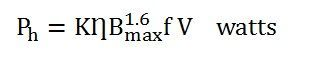# Types of Losses in a Transformer

There are various types of losses in the transformer such as iron loss, copper loss, hysteresis loss, eddy current loss, stray loss, and dielectric loss. The hysteresis losses occur because of the variation of the magnetization in the core of the transformer and the copper loss occurs because of the transformer winding resistance.

The various types of losses are explained below in detail.

Contents:

## Iron Losses

Iron losses are caused by the alternating flux in the core of the transformer as this loss occurs in the core it is also known as Core loss. Iron loss is further divided into hysteresis and eddy current loss.

### Hysteresis Loss

The core of the transformer is subjected to an alternating magnetizing force, and for each cycle of emf, a hysteresis loop is traced out. Power is dissipated in the form of heat known as hysteresis loss and given by the equation shown below:Where

• KȠ is a proportionality constant which depends upon the volume and quality of the material of the core used in the transformer,
• f is the supply frequency,
• Bmax is the maximum or peak value of the flux density.

The iron or core losses can be minimized by using silicon steel material for the construction of the core of the transformer.

### Eddy Current Loss

When the flux links with a closed circuit, an emf is induced in the circuit and the current flows, the value of the current depends upon the amount of emf around the circuit and the resistance of the circuit.

Since the core is made of conducting material, these EMFs circulate currents within the body of the material. These circulating currents are called Eddy Currents. They will occur when the conductor experiences a changing magnetic field. As these currents are not responsible for doing any useful work, and it produces a loss (I2R loss) in the magnetic material known as an Eddy Current Loss.
The eddy current loss is minimized by making the core with thin laminations.

The equation of the eddy current loss is given as:Where,

• Ke – coefficient of eddy current. Its value depends upon the nature of magnetic material like volume and resistivity of core material, the thickness of laminations
• Bm – maximum value of flux density in wb/m2
• T – thickness of lamination in meters
• F – frequency of reversal of the magnetic field in Hz
• V – the volume of magnetic material in m3

## Copper Loss Or Ohmic Loss

These losses occur due to ohmic resistance of the transformer windings. If I1 and I2 are the primary and the secondary current. R1 and R2 are the resistance of primary and secondary winding then the copper losses occurring in the primary and secondary winding will be I12R1 and I22R2 respectively.

Therefore, the total copper losses will beThese losses varied according to the load and known hence it is also known as variable losses. Copper losses vary as the square of the load current.

## Stray Loss

The occurrence of these stray losses is due to the presence of leakage field. The percentage of these losses are very small as compared to the iron and copper losses so they can be neglected.

## Dielectric Loss

Dielectric loss occurs in the insulating material of the transformer that is in the oil of the transformer, or in the solid insulations. When the oil gets deteriorated or the solid insulation gets damaged, or its quality decreases, and because of this, the efficiency of the transformer gets affected.